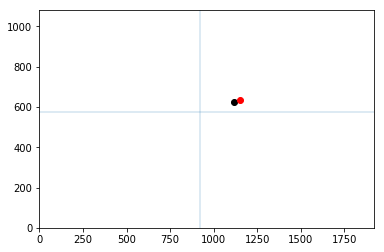Given two image-points from two different positions of a camera, we want to calculate the 3D coordinate that this point is located at in the world. This is highly related to Structure from Motion (sfm) approaches, where we try to create a dense 3d point-cloud using consecutive images (of course this will only work if the 3D worldpoint in question is not moving itself, or if we know it’s movement):In this post I will present two things: the first is how to project a point from known 3d-coordinates to the image plain (2d-coordinates) using the camera-matrix and external transformations (rotation and translation). The second part shows how to use the Direct Linear Transform (DLT) method described in Hartley & Zisserman’s Multiple View Geometry for linear triangulation (this is the method implemented in OpenCV’s triangulatepoints(), so we will use this).

In :
```%matplotlib inline
"""
Test projection and triangulation functions of OpenCV
- Given a camera calibration:
- define two camera poses (rotation and translation)
- compute the projection matrix for each pose
- define some point in 3d
- project it to image coordinates
- Given two camera poses and two projected points:
- use OpenCVs triangulatePoints
(from: HartleyZisserman, Multiple View Geometry, DLT-Linear triangulation)
to retrieve the original 3D points

Axis semantics:
x - right
y - down
z - forward
"""
import math
import cv2
import numpy as np
import matplotlib.pyplot as plt
```
In :
```# camera constants
fl = [1.487847159024568555e+03, 1.489275578039685570e+03]
pp = [9.200624828553764019e+02, 5.759643410906321606e+02]
pitch=0.0
yaw = 0.0
roll = 0.0
```
In :
```def eulerAnglesToRotationMatrix( theta) :
R_x = np.array([[1,         0,                  0                   ],
[0,         math.cos(theta), -math.sin(theta) ],
[0,         math.sin(theta), math.cos(theta)  ]
])

R_y = np.array([[math.cos(theta),    0,      math.sin(theta)  ],
[0,                     1,      0                   ],
[-math.sin(theta),   0,      math.cos(theta)  ]
])

R_z = np.array([[math.cos(theta),    -math.sin(theta),    0],
[math.sin(theta),    math.cos(theta),     0],
[0,                     0,                      1]
])

R = np.dot(R_z, np.dot( R_y, R_x ))

return R
```
In :
```# lets leave rotation and the camera matrix unchanged
R = eulerAnglesToRotationMatrix([0,0,0]) # Rotation matrix:
K = np.array([
[fl, 0., pp],
[0., fl, pp],
[0., 0., 1.]
]) # Kamera matrix:

# define pose 0
T0 = np.array([0,0,0]) # Translation vector
RT0 = np.zeros((3,4))  # combined Rotation/Translation matrix
RT0[:3,:3] = R
RT0[:3, 3] = T0
P0 = np.dot(K, RT0) # Projection matrix

# define pose 1
T1 = np.array([0,0,2.])
RT1 = np.zeros((3,4))
RT1[:3,:3] = R
RT1[:3, 3] = -T1
P1 = np.dot(K, RT1)
```
In :
```wcsp = np.array([2.0, 0.5, 15., 1.]) # define an arbitrary 3D point in world coordinates

icsp0 = np.dot(P0, wcsp) # project this point using the first camera pose
icsp0 = icsp0/icsp0[-1] # normalize as we are in homogenuous coordinates

icsp1 = np.dot(P1, wcsp)
icsp1 = icsp1/icsp1[-1]

# plot the projection
fig = plt.figure()
plt.xlim((0,1920))
plt.ylim((0,1080))

plt.plot(icsp0, icsp0, 'ko')
plt.plot(icsp1, icsp1, 'ro')

plt.axvline(pp, alpha=0.2)
plt.axhline(pp, alpha=0.2)
plt.show()
```In :
```res = cv2.triangulatePoints(P0, P1, icsp0[:2], icsp1[:2]) # now try to get those 3d-positions back using triangulation
print "Abracadabra, are these the coordinates you put in the hat?"
print res[:3]/res[-1] # again, normalize b/c of homogenuous coordinates
```
```Abracadabra, are these the coordinates you put in the hat?
[[ 2. ]
[ 0.5]
[15. ]]
```
TobiasWeis | 14. April 2018